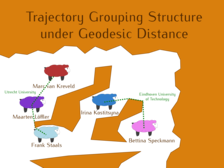Trajectory Grouping Structure under Geodesic Distance

AbstractIn recent years trajectory data has become one of the main types of geographic data, and hence algorithmic tools to handle large quantities of trajectories are essential. A single trajectory is typically represented as a sequence of time-stamped points in the plane. In a collection of trajectories one wants to detect maximal groups of moving entities and their behaviour (merges and splits) over time. This information can be summarized in the trajectory grouping structure.

Significantly extending the work of Buchin et al. [JoCG 2015] into a realistic setting, we show that the trajectory grouping structure can be computed efficiently also if obstacles are present and the distance between the entities is measured by geodesic distance. We bound the number of critical events: times at which the distance between two subsets of moving entities is exactly $$\varepsilon$$, where $$\varepsilon$$ is the threshold distance that determines whether two entities are close enough to be in one group. In case the $$n$$ entities move in a simple polygon along trajectories with $$\tau$$ vertices each we give an $$O(\tau n^2)$$ upper bound, which is tight in the worst case. In case of well-spaced obstacles we give an $$O(\tau(n^2 + m\lambda_4(n)))$$ upper bound, where $$m$$ is the total complexity of the obstacles, and $$\lambda_s(n)$$ denotes the maximum length of a Davenport-Schinzel sequence of $$n$$ symbols of order $$s$$. In case of general obstacles we give an $$O(\tau\min\{n^2 + m^3\lambda_4(n), n^2m^2\})$$ upper bound. Furthermore, for all cases we provide efficient algorithms to compute the critical events, which in turn leads to efficient algorithms to compute the trajectory grouping structure.

Corresponding Publications

• Trajectory Grouping Structure under Geodesic Distance

Proc. 31th Annual Symposium on Computational Geometry, 2015
@inproceedings{geogrouping2015,
author = {Kostitsyna, Irina and van Kreveld, Marc and L{\"o}ffler, Maarten and
Speckmann, Bettina and Staals, Frank},
title = {Trajectory Grouping Structure under Geodesic Distance},
booktitle = {Proc. 31th Annual Symposium on Computational Geometry},
year = {2015},
location = {Eindhoven, The Netherlands},
numpages = {15},
keywords = {trajectories, grouping, moving entity, geodesic distance},
category = {trajectories},
pages = {674--688},
series = {Leibniz International Proceedings in Informatics (LIPIcs)},
isbn = {978-3-939897-83-5},
issn = {1868-8969},
volume = {34},
publisher = {Schloss Dagstuhl--Leibniz-Zentrum fuer Informatik},
url = {https://dx.doi.org/10.4230/LIPIcs.SOCG.2015.674},
doi = {10.4230/LIPIcs.SOCG.2015.674},
}

• New Results on Trajectory Grouping under Geodesic Distance

Abstr. 32th European Workshop on Computational Geometry (EuroCG), 2016
@article{geodesic_extras_eurocg2016,
author = {L{\"o}ffler, Maarten and Staals, Frank and Urhausen, J{\'e}r{\^o}me},
title = {New Results on Trajectory Grouping under Geodesic Distance},
journal = {Abstr. 32th European Workshop on Computational Geometry (EuroCG)},
year = {2016},
location = {Lugano, Switzerland},
numpages = {4},
category = {trajectories},
project = {geogrouping2015},
url = {http://www.eurocg2016.usi.ch/sites/default/files/paper_70.pdf},
}